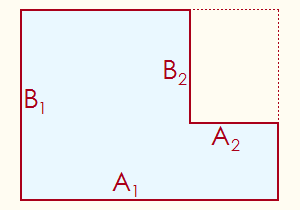# How to Figure the Area and Perimeter of an L-Shaped Room

## L-Shape Area and Perimeter Calculator

L-Shaped Room Calculator
Outer L Width ft.
Outer L Length ft.
Inner L Width ft.
Inner L Length ft.Many homes contain rooms that are shaped like an L rather than a perfect rectangle. If you need to know the area and perimeter of an L-shaped room for remodeling purposes, you can calculate these measures easily by hand or with the calculator on the left.

An L-shape has six sides, five concave corners, and one convex corner. For purposes of calculation, you only need to know the width and length of the outer part of the L, and the width and length of the inner part of the L. For the formulas below, A1 and B1 represent the outer width and length respectively, and A2 and B2 represent the inner width and length respectively. See image on the left.

The area of an L-shaped room is given by the formula

Area = A1B1 - A2B2.

The perimeter of an L-shaped room is given by the formula

Perimeter = 2(A1 + B1).

Notice that the perimeter does not depend on the values of A2 and B2.